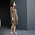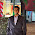VHDL coding tips and tricks: Generating random numbers in a VHDL testbench

## Thursday, August 15, 2013

### Generating random numbers in a VHDL testbench

I have written two posts about random number generation in vhdl before. But these were written from a synthesisable point of view. So they are a bit complex. But if you are looking just for a simulatable code( for example to be used in a testbench) then there is a much simpler way to generate random numbers.

We use the procedure named UNIFORM which is defined in the IEEE library named math_real. Without too much explanation I will share the code with you.

library ieee;
use ieee.math_real.all;

entity rand_gen is
end rand_gen;

architecture behavior of rand_gen is

signal rand_num : integer := 0;

begin

process
variable seed1, seed2: positive;               -- seed values for random generator
variable rand: real;   -- random real-number value in range 0 to 1.0
variable range_of_rand : real := 1000.0;    -- the range of random values created will be 0 to +1000.
begin
uniform(seed1, seed2, rand);   -- generate random number
rand_num <= integer(rand*range_of_rand);  -- rescale to 0..1000, convert integer part
wait for 10 ns;
end process;

end behavior;

The code generates random numbers with a time gap of 10 ns between two successive numbers. The variable named range_of_rand defines how big the random number can get. The uniform procedure generates numbers between 0.0 and 1.0. So to generate big numbers you just multiply it by a real number( in the above code 1000.0 ) and type cast it to an integer.

Using functions like conv_std_logic_vector or to_unsigned you can convert these random integers into binary format vectors. Remember to declare the required additional libraries in such cases.

Note :- The code was simulated using Xilinx ISE 13.1 Webpack. It should be able to work in other

#### 8 comments:

1.hey, im a bit new to VHDL. Is this code suppose to be in the test bench file?

2.It is generating an error "Nonconstant REAL value is not supported for synthesis" . What should I do?

3.I am getting an error "Nonconstant REAL value is not supported for synthesis"

1.This is not synthesisable code, just use it for testbenches and simulation not for synthesis. This is clearly stated in the article ...

4.https://cdn.meme.am/instances/400x/66689217.jpg

5.Hey, what changes should i made in this code to generate Binary random numbers ?

1.Check,linear-feedback shift register (LFSR). U can generate pseudo-random numbers by using this algorithm.

6.Bro how can I use this to simulate a dice game